# Tutorial How to Place Multiple Ad Units In The Middle ArticlesKlick EditHTML will look like this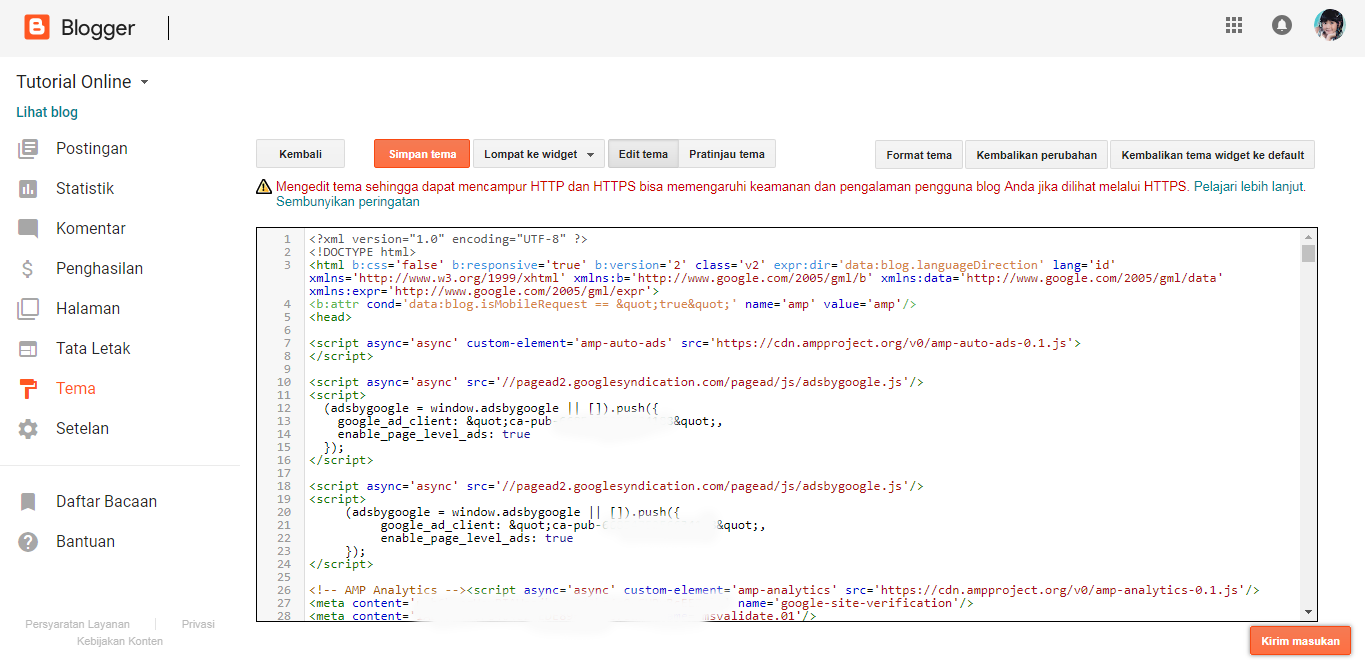Please search the code data:post.body/ For easy searching use the keyboard key combination CTRL + F then it will appear in the top right corner of the search field as the following figureCopy The code we will be looking for above then Pastap on the search field Enter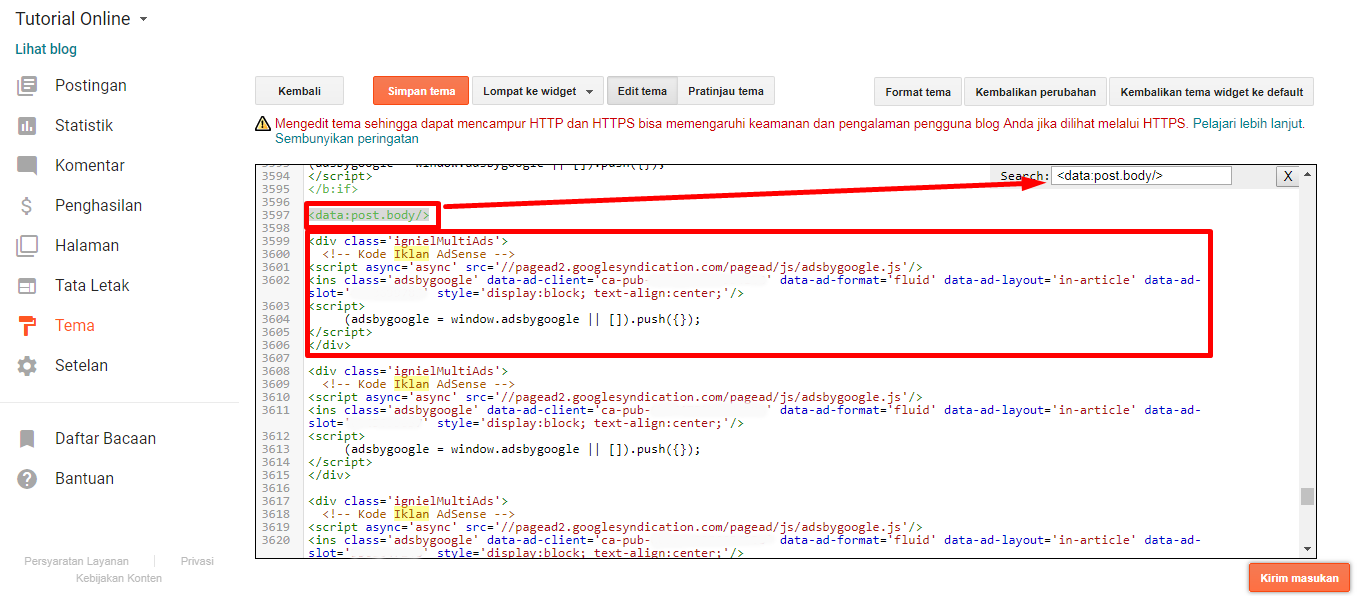If the code search results data:post.body/ More than one then specify the code that is in the conditional tag area of the posting page. In the Admin template, the code in question is in the position when the search results. If you have met the following code pas press:
``````
</div>
``````

Click on new ad units to create an In Article type adIn the new ad, Unit form select InArticle AD as shown in the above Tambar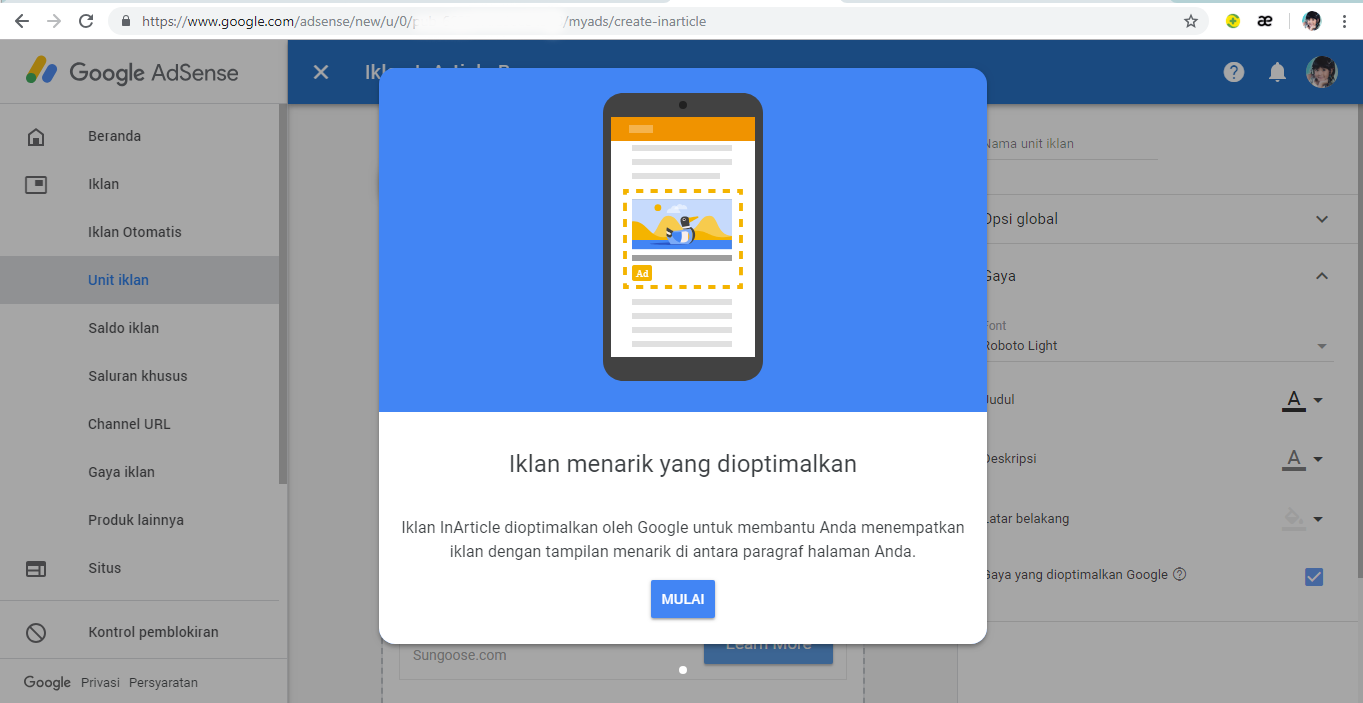Klick start to continuePlease do not forget to enter the ad name in the column "ad Unit name" as shown belowAfter finishing setting banner ad display, click Save and get codePaste In The Adsense ad code Article below <div class='ignielMultiAds'> or under<!-- Kode Iklan AdSense -->The result as below screenshot There are 3 ad codes in a row because Admin put 3 ads in 1 article is considered enough. Her ads will appear separately automatically yes blogger bloggers even if the code is sequential later the display on the article will be separate because the ads appear automatically based on the number of paragraphs available in the Post article.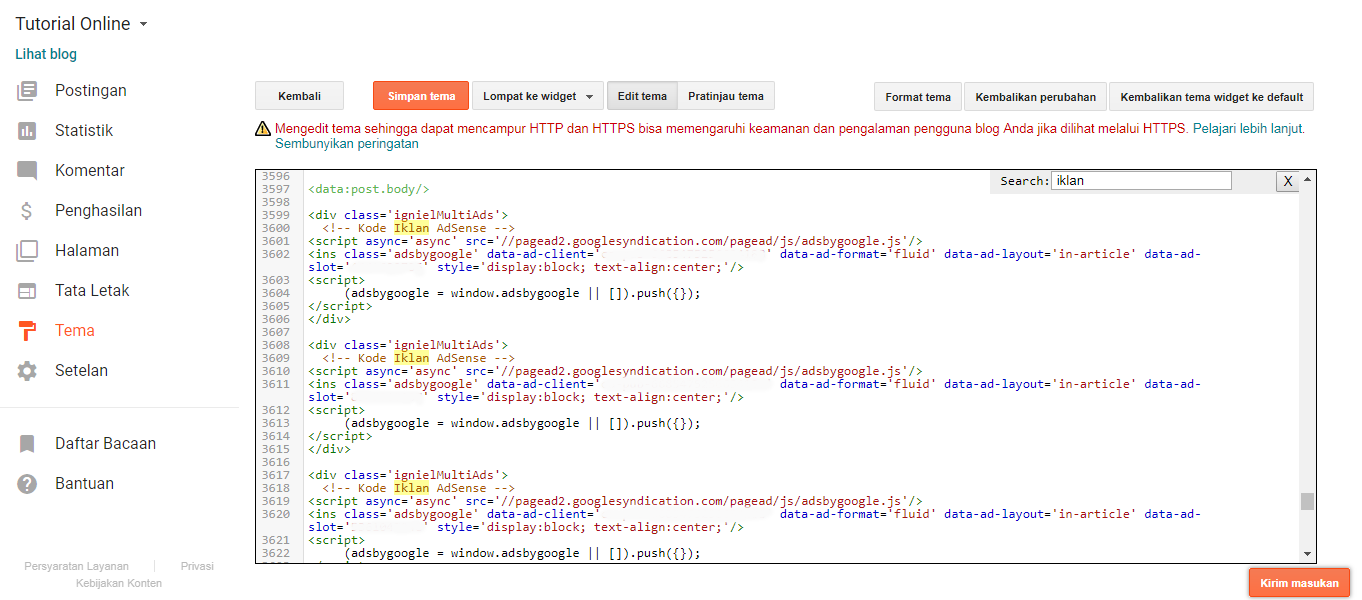If Blog Buddy blogger dominated the article with a lengthy discussion until it takes several times Scroll Tuesday, down to read his articles then the number of advertisements can be increased to 4, 5 or 6 ads in one article.

But if the article post blogger Buddy is dominated article with a discussion that brushes admins recommend not more than 3 ads because it will impress the ad pile. Install 2 or 3 ads only.

Now search Code </body> Like the way above Copypaste in the search field then press EnterIf it has been found the position of the code past press the following codes exactly above the </body>code

`````` <b:if cond='data:view.isPost'>
<script> //<![CDATA[
// Multiple Ads Inside Post by igniel.com
eval(function(p,a,c,k,e,d){e=function(c){return(c<a?'':e(parseInt(c/a)))+((c=c%a)>35?String.fromCharCode(c+29):c.toString(36))};if(!''.replace(/^/,String)){while(c--){d[e(c)]=k[c]||e(c)}k=[function(e){return d[e]}];e=function(){return'\\w+'};c=1};while(c--){if(k[c]){p=p.replace(new RegExp('\\b'+e(c)+'\\b','g'),k[c])}}return p}('N a=["\\v\\i\\r\\l\\i\\b\\f\\T\\B\\f\\g\\i\\A\\o\\n","\\S\\B\\b\\j\\w\\y\\b\\f\\b\\D\\g\\e\\j\\A\\f\\f","\\v\\q\\e\\n\\g\\C\\t\\e\\o\\w\\u\\t\\j\\U\\u\\v\\q\\e\\n\\g\\C\\t\\e\\o\\w\\u\\q","\\f\\b\\l\\r\\g\\Q","\\z\\j\\e\\F","\\n\\f\\i\\D\\b","\\f\\e\\r","\\l\\e\\o\\b\\G\\E\\F\\b","\\M","\\i\\l\\n\\b\\j\\g\\O\\b\\z\\e\\j\\b","\\q\\E\\j\\b\\l\\g\\G\\e\\o\\b","\\l\\b\\Y\\g\\y\\i\\t\\f\\i\\l\\r"];(13(){s p=H[a](a);L(p){s h=H[a](a),x=p[a]+1,K=h[a]/x;c=Z[a]({X:x},(12,I)=>I+1),d=c[a](0,-1);W(s m=0;m<d[a];m++){s J=d[m],k=V((K*J));P[a](h[k]);L(h[k][a]==a){h[k][a][a](p[m],h[k])}R{h[k][a][a](p[m],h[k][a])}}}})()',62,66,'||||||||||_0xa93d|x65|||x6F|x6C|x74|_0x51c1x2|x69|x72|_0x51c1x9|x6E|_0x51c1x7|x73|x64|_0x51c1x1|x70|x67|let|x62|x20|x2E|x79|_0x51c1x3|x53|x66|x41|x75|x2D|x63|x61|x6D|x4E|document|_0x51c1x6|_0x51c1x8|_0x51c1x4|if|x50|var|x42|console|x68|else|x71|x4D|x2C|parseInt|for|length|x78|Array|||_0x51c1x5|function'.split('|'),0,{}));
//]]> </script>
</b:if>``````

The result is as shown below.Then search the code <b>/style</b> or <b>]]></b:skin</b> Add the following CSS code to make the ad less tightly related to the post content above and below.
``````
/* Multiple Ads Inside Post by igniel.com */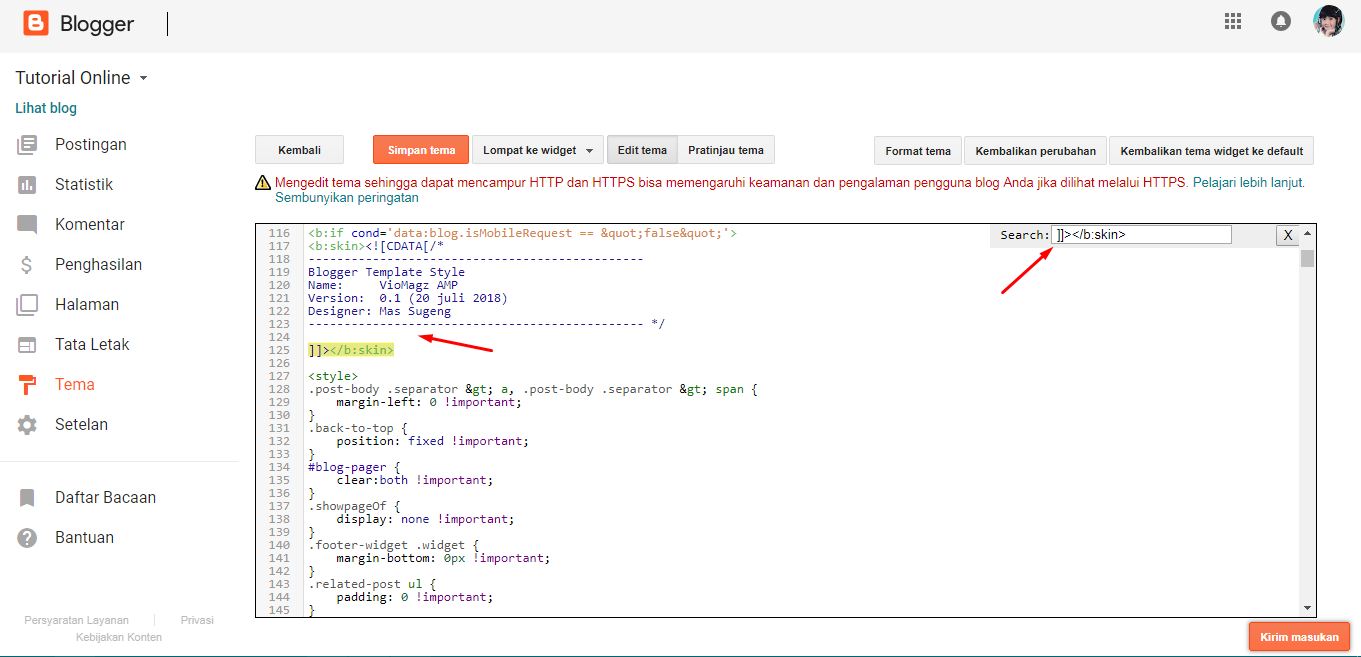Place it above the code <b>/style or ]]></b:skin</b> Result as below screenshot shownThe last step saves and sees the results Now Buddy bloggers already have advertisements that are displayed in the middle of the post, congratulations hopefully can increase the number of ads Klick.

Such a tutorial on how to place multiple AdSense ads in a blog post article, this tutorial can also be applied to other advertising publishers so do not have to be from Google Adsense. For a blogger who doesn't have an AdSense, an account could implement another ad with a banner ad code corresponding to an existing ad publisher. Thank you for listening to this tutorial until it is complete, hopefully, useful and see you in a later tutorial.